﻿ 基于支路可用容量熵的概率静态安全分析 Probabilistic Static Security Analysis Based on Entropy of Branch Available Capacity

Transmission and Distribution Engineering and Technology
Vol.06 No.04(2017), Article ID:23164,9 pages

Probabilistic Static Security Analysis Based on Entropy of Branch Available Capacity

Jie Yu1, Min Sun2, Peng Li3, Wei Zeng2, Saite Yang4

1School of Electrical Engineer, Southeast University, Nanjing Jiangsu

2State Grid Jiangxi Power Company Institute, Nanchang Jiangxi

3Nanjing Guodian Nanzi Power Grid Automation Co. Ltd., Nanjing Jiangsu

4College of Energy and Electrical Engineering, Hohai University, Nanjing Jiangsu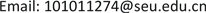Received: Dec. 1st, 2017; accepted: Dec. 16th, 2017; published: Dec. 26th, 2017ABSTRACT

For the power grid with large-scale wind power integration, using the probabilistic power flow calculation and the concept of entropy, a ranking indicator based on the available capacity entropy of branch and sorting method under multi-scenarios for expected contingency are proposed in order to speed up the probabilistic static security analysis and the impact of branch outage on the system can be described in the view of branch available capacity. The simulation is verified in IEEE-118 system and the results indicate that the proposed method can evaluate power system static security precisely and find the weakness of power system. Meanwhile, it greatly shortens the time and provides reference information for electric power operation personnel.

Keywords:Entropy of Branch Available Capacity, Probabilistic Power Flow, Static Security Analysis, Expected Contingency Sorting

1东南大学电气工程学院，江苏 南京

2国网江西省电力公司电力科学研究院，江西 南昌

3南京国电南自电网自动化有限公司，江苏 南京

4河海大学能源与电气学院，江苏 南京1. 引言

2. 基于支路可用容量熵的排序指标

$H=-C\underset{i=1}{\overset{m}{\sum }}P\left({X}_{i}\right)\mathrm{ln}P\left({X}_{i}\right)$ (1)

${r}_{ki}=\frac{{C}_{k}-{\alpha }_{ki}}{{C}_{k}}$ (2)

${\beta }_{ki}={r}_{ki}/\underset{\begin{array}{l}k=1\\ k\ne i\end{array}}{\overset{n}{\sum }}{r}_{ki}$ (3)

${H}_{Ci}=-\underset{\begin{array}{l}k=1\\ k\ne i\end{array}}{\overset{n}{\sum }}{\beta }_{ki}\mathrm{ln}{\beta }_{ki}$ (4)

${I}_{i}=\frac{{E}_{i}}{{H}_{Ci}}$ (5)

1) 支路 $i$ 基态下有功功率的期望值 ${E}_{i}$ 越大，开断该支路后其有功向附近其他支路进行转移对其他支路有功的影响越大；

2) 开断支路 $i$ 后得到的支路可用容量熵 ${H}_{Ci}$ 越小，支路 $i$ 的有功转移分布越聚集在少数部分的几条支路上，使得部分支路可用容量率很小，处于重载甚至过载状态，越限概率很高。

3. 基于多场景下的排序方法

1) 利用拉丁超立方抽样抽取每个风电场输出功率的样本值，数量为N，即场景数。

2) 根据风电场出力的样本值，在每一个场景下计算系统内各支路基态下的有功功率，并对所有支路一一进行开断，通过直流潮流计算出除开断支路以外所有支路的有功功率，直至所有场景下所有支路都完成了潮流计算。

3) 根据得到的潮流结果计算出所有预想事故基于支路可用容量熵的排序指标，对系统造成的影响程度由大到小进行排列，得到预想事故一览表。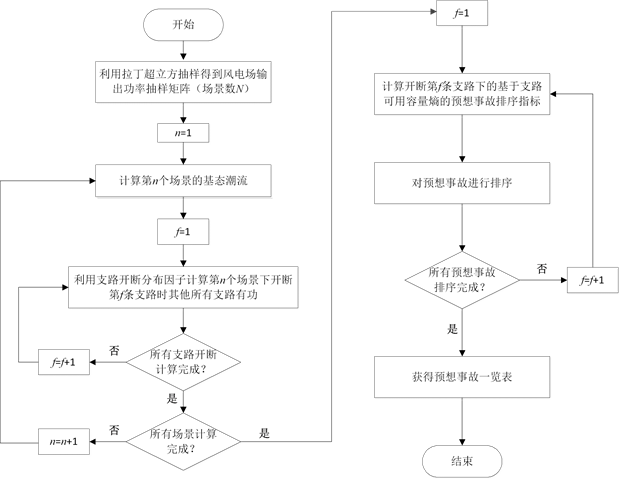Figure 1. Flow chart of contingency accident sequencing method, based on multiple scenes

4. 基于支路可用容量熵的概率静态安全分析

1) 对系统内所有支路依次进行开断进行直流概率潮流分析，根据预想事故排序指标快速得到预想事故一览表；

2) 根据表中的预想事故顺序，依次进行交流概率潮流分析，计算支路有功功率的期望、方差、越限概率和概率分布等结果，进一步获得电力系统静态安全概率评价指标；

3) 如果满足终止判据则跳出循环，即连续校验5个预想事故均不引起系统内其他支路有功功率越限，否则转至步骤2)。

5. 算例分析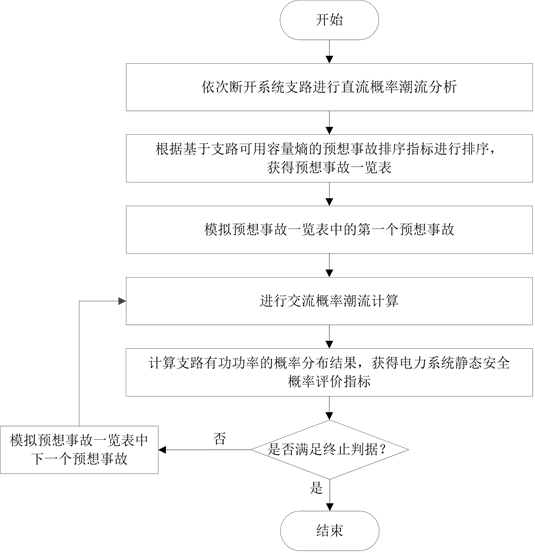Figure 2. Flow chart of static security analysis, based on probabilistic load flow

$PR{I}_{i}=\underset{\begin{array}{l}k=1\\ k\ne i\end{array}}{\overset{n}{\sum }}P\left(|{p}_{ki}|>{p}_{k\mathrm{max}}\right)$ ，其中 ${p}_{ki}$ 为开断支路 $i$ 后，支路 $k$ 的有功功率  。阈值设为0.001，即当

$PR{I}_{i}<0.001$ 时，认为该事故不会引起系统过负荷。其中，阈值的设定基于系统结构以及系统状态的变化。经过大量实验数据的校验，将阈值设置为0.001时，据此阈值判断出的事故情况最符合实际系统发生事故时的情况。开断预想事故的静态安全概率评价指标计算结果如表2所示。Table 2. Evaluation index of the static security probabilityTable 3. Limit violation probability of some lines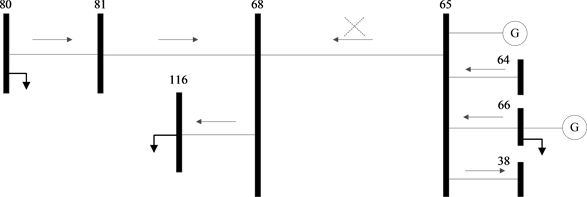Figure 3. Network structure diagram near line 65-68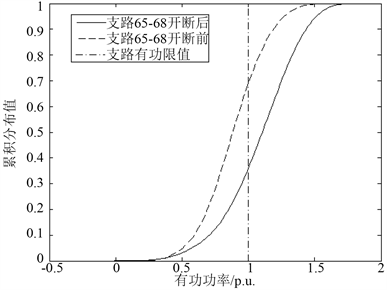Figure 4. Active power cumulative distribution curve of line 68-81 before and after line 65-69 outage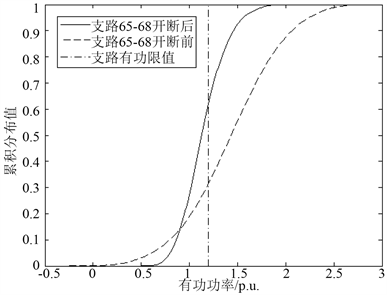Figure 5. Active power cumulative distribution curve of line 64-65 before and after line 65-68 outage

6. 结束语

Probabilistic Static Security Analysis Based on Entropy of Branch Available Capacity[J]. 输配电工程与技术, 2017, 06(04): 55-63. http://dx.doi.org/

1. 1. 留毅, 章静芳. 电力系统静态安全分析综述[J]. 科技创新导报, 2010(30): 64-64.

2. 2. 朱星阳, 黄宇峰, 张建华, 等. 基于随机潮流的含风电电力系统静态安全评估[J]. 电力系统自动化, 2014, 38(20): 46-53.

3. 3. 傅旭. 考虑不确定因素的电力系统静态安全预防控制[J]. 电力自动化设备, 2014, 34(2): 120-124.

4. 4. 张志坚, 乔振宇, 王建东, 等. 预想事故排序算法比较[J]. 电力自动化设备, 2001, 21(11): 25-27.

5. 5. 马平, 蔡兴国. 电压稳定分析中支路型故障筛选及排序算法研究[J]. 中国电机工程学报, 2007, 27(1): 44-48.

6. 6. 邱晓燕, 李兴源, 林伟. 在线电压稳定性评估中事故筛选和排序方法的研究[J]. 中国电机工程学报, 2004, 24(9): 50-55.

7. 7. 王永辉, 王克文, 刘畅, 等. 概率潮流在电力系统静态N-1校验中的应用[J]. 计算机仿真, 2013, 30(2): 125-129.

8. 8. 陈波, 郭勇, 施世鸿, 等. 一种预想事故自动选择的新方法[J]. 陕西电力, 2009, 37(5): 10-14.

9. 9. 倪涌炯, 方鸽飞, 叶剑锋. 基于电压稳定的在线预想事故筛选和排序[J]. 电工技术, 2006(2): 31-32.

10. 10. 苟竞, 刘俊勇, 刘友波, 等. 基于能量熵测度的电力系统连锁故障风险辨识[J]. 电网技术, 2013, 37(10): 2754-2761.

11. 11. 李勇, 刘俊勇, 刘晓宇, 等. 基于潮流熵的电网连锁故障传播元件的脆弱性评估[J]. 电力系统自动化, 2012, 36(19): 11-16.

12. 12. 曹娟, 张颖淳, 苏伯洪. 基于流量熵的道路交通网络脆弱性优化[J]. 西南师范大学学报(自然科学版), 2014(7): 30-35.

13. 13. 刘文颖, 王佳明, 谢昶, 等. 基于脆性风险熵的复杂电网连锁故障脆性源辨识模型[J]. 中国电机工程学报, 2012, 32(31): 142-149.

14. 14. 杜正旺, 哈恒旭, 宋扬, 等. 基于灵敏度–补偿法的电力网络开断潮流新算法[J]. 电力系统保护与控制, 2010, 38(16): 103-107.

15. 15. 刘怡芳, 张步涵, 李俊芳, 等. 考虑电网静态安全风险的随机潮流计算[J]. 中国电机工程学报, 2011(1): 59-64.

16. 16. 蒋平, 杨绍进, 霍雨翀. 考虑风电场出力随机性的电网静态安全分析[J]. 电力系统自动化, 2013, 37(22): 35-40.